Need help? Email help@shout.education

# Atomic Orbitals

This page explains what atomic orbitals are in a way that makes them understandable for introductory courses such as UK A-level and its equivalents. It explores s and p orbitals in some detail, including their shapes and energies. d orbitals are described only in terms of their energy, and f orbitals only get a passing mention.

## What is an Atomic Orbital?

### Orbitals and Orbits

When a planet moves around the sun, you can plot a definite path for it which is called an orbit. A simple view of the atom looks similar and you may have pictured the electrons as orbiting around the nucleus. The truth is different, and electrons in fact inhabit regions of space known as orbitals.

Orbits and orbitals sound similar, but they have quite different meanings. It is essential that you understand the difference between them.

### The impossibility of Drawing Orbits for Electrons

To plot a path for something you need to know exactly where the object is and be able to work out exactly where it's going to be an instant later. You can't do this for electrons.

The Heisenberg Uncertainty Principle says – loosely – that you can't know with certainty both where an electron is and where it's going next. (What it actually says is that it is impossible to define with absolute precision, at the same time, both the position and the momentum of an electron.)

That makes it impossible to plot an orbit for an electron around a nucleus. Is this a big problem? No. If something is impossible, you have to accept it and find a way around it.

Note: Over the years I have had a steady drip of questions from students in which it is obvious that they still think of electrons as orbiting around a nucleus – which is completely wrong! I have added a page about why the idea of orbits is wrong to try to avoid having to say the same thing over and over again!

### Hydrogen's Electron – the 1s Orbital

Note: In this diagram (and the orbital diagrams that follow), the nucleus is shown very much larger than it really is. This is just for clarity.Suppose you had a single hydrogen atom and at a particular instant plotted the position of the one electron. Soon afterwards, you do the same thing, and find that it is in a new position. You have no idea how it got from the first place to the second.

You keep on doing this over and over again, and gradually build up a sort of 3D map of the places that the electron is likely to be found.

In the hydrogen case, the electron can be found anywhere within a spherical space surrounding the nucleus. The diagram shows a cross-section through this spherical space.

95% of the time (or any other percentage you choose), the electron will be found within a fairly easily defined region of space quite close to the nucleus. Such a region of space is called an orbital. You can think of an orbital as being the region of space in which the electron lives.

Note: If you wanted to be absolutely 100% sure of where the electron is, you would have to draw an orbital the size of the Universe!

What is the electron doing in the orbital? We don't know, we can't know, and so we just ignore the problem! All you can say is that if an electron is in a particular orbital it will have a particular definable energy.

Each orbital has a name.

The orbital occupied by the hydrogen electron is called a 1s orbital. The "1" represents the fact that the orbital is in the energy level closest to the nucleus. The "s" tells you about the shape of the orbital. s orbitals are spherically symmetric around the nucleus – in each case, like a hollow ball made of rather chunky material with the nucleus at its centre.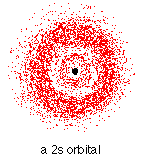The orbital on the left is a 2s orbital. This is similar to a 1s orbital except that the region where there is the greatest chance of finding the electron is further from the nucleus – this is an orbital at the second energy level.

If you look carefully, you will notice that there is another region of slightly higher electron density (where the dots are thicker) nearer the nucleus. ("Electron density" is another way of talking about how likely you are to find an electron at a particular place.)

2s (and 3s, 4s, etc) electrons spend some of their time closer to the nucleus than you might expect. The effect of this is to slightly reduce the energy of electrons in s orbitals. The nearer the nucleus the electrons get, the lower their energy.

3s, 4s (etc) orbitals get progressively further from the nucleus.

### p OrbitalsNot all electrons inhabit s orbitals (in fact, very few electrons live in s orbitals). At the first energy level, the only orbital available to electrons is the 1s orbital, but at the second level, as well as a 2s orbital, there are also orbitals called 2p orbitals.

A p orbital is rather like 2 identical balloons tied together at the nucleus. The diagram on the left is a cross-section through that 3-dimensional region of space. Once again, the orbital shows where there is a 95% chance of finding a particular electron.

Taking chemistry further: If you imagine a horizontal plane through the nucleus, with one lobe of the orbital above the plane and the other beneath it, there is a zero probability of finding the electron on that plane. So how does the electron get from one lobe to the other if it can never pass through the plane of the nucleus? At this introductory level you just have to accept that it does! If you want to find out more, read about the wave nature of electrons.

Unlike an s orbital, a p orbital points in a particular direction – the one drawn points up and down the page.

At any one energy level it is possible to have three absolutely equivalent p orbitals pointing mutually at right angles to each other. These are arbitrarily given the symbols px, py and pz. This is simply for convenience – what you might think of as the x, y or z direction changes constantly as the atom tumbles in space.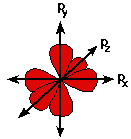The p orbitals at the second energy level are called 2px, 2py and 2pz. There are similar orbitals at subsequent levels – 3px, 3py, 3pz, 4px, 4py, 4pz> and so on.

All levels except for the first level have p orbitals. At the higher levels the lobes get more elongated, with the most likely place to find the electron more distant from the nucleus.

### d and f Orbitals

In addition to s and p orbitals, there are two other sets of orbitals which become available for electrons to inhabit at higher energy levels. At the third level, there is a set of five d orbitals (with complicated shapes and names) as well as the 3s and 3p orbitals (3px, 3py, 3pz). At the third level there are a total of nine orbitals altogether.

At the fourth level, as well the 4s and 4p and 4d orbitals there are an additional seven f orbitals – 16 orbitals in all. s, p, d and f orbitals are then available at all higher energy levels as well.

For the moment, you need to be aware that there are sets of five d orbitals at levels from the third level upwards, but you probably won't be expected to draw them or name them. Apart from a passing reference, you won't come across f orbitals at all.

Note: Some UK-based syllabuses will eventually want you to be able to draw, or at least recognise, the shapes of d orbitals. I am not including them now because I don't want to add confusion to what is already a difficult introductory topic. Check your syllabus and past papers to find out what you need to know. If you are a studying a UK-based syllabus and haven't got these, follow this link to find out how to get hold of them.

## Fitting Electrons into Orbitals

You can think of an atom as a very bizarre house (like an inverted pyramid!) – with the nucleus living on the ground floor, and then various rooms (orbitals) on the higher floors occupied by the electrons. On the first floor there is only 1 room (the 1s orbital); on the second floor there are 4 rooms (the 2s, 2px, 2py and 2pz orbitals); on the third floor there are 9 rooms (one 3s orbital, three 3p orbitals and five 3d orbitals); and so on. But the rooms aren't very big. Each orbital can only hold 2 electrons.

A convenient way of showing the orbitals that the electrons live in is to draw "electrons-in-boxes".

### "Electrons-in-boxes"

Orbitals can be represented as boxes with the electrons in them shown as arrows. Often an up-arrow and a down-arrow are used to show that the electrons are in some way different.

Taking chemistry further: The need to have all electrons in an atom different comes out of quantum theory. If they live in different orbitals, that's fine – but if they are both in the same orbital there has to be some subtle distinction between them. Quantum theory allocates them a property known as "spin" – which is what the arrows are intended to suggest.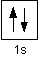A 1s orbital holding 2 electrons would be drawn as shown on the right, but it can be written even more quickly as 1s2. This is read as "one s two" – not as "one s squared".

You mustn't confuse the two numbers in this notation: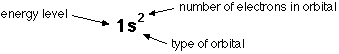### The Order of Filling Orbitals – The Aufbau Principle

Aufbau is a German word meaning building up or construction. We imagine that as you go from one atom to the next in the Periodic Table, you can work out the electronic structure of the next atom by fitting an extra electron into the next available orbital.

Electrons fill low energy orbitals (closer to the nucleus) before they fill higher energy ones. Where there is a choice between orbitals of equal energy, they fill the orbitals singly as far as possible.

This filling of orbitals singly where possible is known as Hund's rule. It only applies where the orbitals have exactly the same energies (as with p orbitals, for example), and helps to minimise the repulsions between electrons and so makes the atom more stable.

The diagram (not to scale) summarises the energies of the orbitals up to the 4p level that you will need to know when you are using the Aufbau Principle.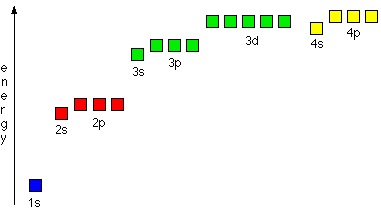Notice that the s orbital always has a slightly lower energy than the p orbitals at the same energy level, so the s orbital always fills with electrons before the corresponding p orbitals.

The real oddity is the position of the 3d orbitals. They are at a slightly higher level than the 4s – and so it is the 4s orbital which you fill first, followed by all the 3d orbitals and then the 4p orbitals.

Similar confusion occurs at higher levels, with so much overlap between the energy levels that you don't fill the 4f orbitals until after the 6s, for example.

For UK-based exam purposes, you simply have to remember that when you are using the Aufbau Principle, you fill the 4s orbital before the 3d orbitals. The same thing happens at the next level as well – you fill the 5s orbital before the 4d orbitals. All the other complications are beyond the scope of this site.

Knowing the order of filling is central to understanding how to write electronic structures. Follow the link below to find out how to do this.